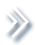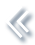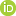####ArchivesReview Articles

## Multi-outcome longitudinal small area estimation – a case study

Eric Slud ,

Center for Statistical Research & Methodology, Census Bureau, Washington, DC, USA; Mathematics Department, University of Maryland, College Park, MD, USAevs@math.umd.edu

Yves Thibaudeau

Center for Statistical Research & Methodology, Census Bureau, Washington, DC, USAPages 136-149 | Received 29 Jan. 2019, Accepted 16 Sep. 2019, Published online: 26 Sep. 2019,
• Abstract
• References
• Citations

ABSTRACT

A recent paper [Thibaudeau, Slud, and Gottschalck (2017). Modeling log-linear conditional probabilities for estimation in surveys. The Annals of Applied Statistics11, 680–697] proposed a ‘hybrid’ method of survey estimation combining coarsely cross-classified design-based survey-weighted totals in a population with loglinear or generalised-linear model-based conditional probabilities for cells in a finer cross-classification. The models were compared in weighted and unweighted forms on data from the US Survey of Income and Program Participation (SIPP), a large national longitudinal survey. The hybrid method was elaborated in a book-chapter [Thibaudeau, Slud, & Cheng (2019). Small-area estimation of cross-classified gross flows using longitudinal survey data. In P. Lynn (Ed.), Methodology of longitudinal surveys II. Wiley] about estimating gross flows in (two-period) longitudinal surveys, by considering fixed versus mixed effect versions of the conditional-probability models and allowing for 3 or more outcomes in the later-period categories used to define gross flows within generalised logistic regression models. The methodology provided for point and interval small-area estimation, specifically area-level two-period labour-status gross-flow estimation, illustrated on a US Current Population Survey (CPS) dataset of survey respondents in two successive months in 16 states. In the current paper, that data analysis is expanded in two ways: (i) by analysing the CPS dataset in greater detail, incorporating multiple random effects (slopes as well as intercepts), using predictive as well as likelihood metrics for model quality, and (ii) by showing how Bayesian computation (MCMC) provides insights concerning fixed- versus mixed-effect model predictions. The findings from fixed-effect analyses with state effects, from corresponding models with state random effects, and fom Bayes analysis of posteriors for the fixed state-effects with other model coefficients fixed, all confirm each other and support a model with normal random state effects, independent across states.

## References

1. Agresti, A. (2013). Categorical data analysis. Hoboken, NJ: Wiley. [Google Scholar]
2. Bickel, P., & Doksum, K. (2015). Mathematical statistics: Basic ideas and slected topics (2nd ed. Vol. I). Boca Raton, FL: Chapman & Hall/CRC. [Google Scholar]
3. Casella, G., & Robert, C. (2005). Monte Carlo statistical methods (2nd Ed.). New York: Springer. [Google Scholar]
4. Fienberg, S. (1980). The measurement of crime victimization: Prospects for a panel analysis of a panel survey. Journal of the Royal Statistical Society Series D29, 313–350. [Google Scholar]
5. Gelman, A., & Hill, J. (2007). Data analysis using regression and multilevel/hierarchical models. New York: Cambridge Univ. Press. [Google Scholar]
6. Hand, D., & Till, R. (2001). A simple generalization of the area under the ROC curve for multiple class classification problems. Machine Learning45(2), 171–186. doi: 10.1023/A:1010920819831 [Crossref][Web of Science ®], [Google Scholar]
7. Li, J., & Fine, J. (2008). ROC analysis with multiple classes and multiple tests: Methodology and its application in microarray studies. Biostatistics9, 566–576. Retrieved from https://doi.org/10.1093/biostatistics/kxm050 [Crossref][Web of Science ®], [Google Scholar]
8. Pfeffermann, D., Skinner, C., & Humphreys, K. (1998). The estimation of gross flows in the presence of measurement error using auxiliary variables. Journal of the Royal Statistical Society Series A161, 13–32. doi: 10.1111/1467-985X.00088 [Crossref][Web of Science ®], [Google Scholar]
9. Pinheiro, J., & Bates, D. (1995). Approximations of the loglikelihood function in the nonlinear mixed effects model. Journal of Computational and Graphical Statistics4, 12–35. [Taylor & Francis Online], [Google Scholar]
10. Rao, J. N. K, & Molina, I. (2015). Small area estimation (2nd ed.). Hoboken, NJ: John Wiley. [Crossref][Google Scholar]
11. R Core Team (2017). R: A language and environment for statistical computing. R Foundation for Statistical Computing, Vienna, Austria. Retrieved from https://www.R-project.org/ [Google Scholar]
12. Särndal, C.-E., Swensson, J., & Wretman, J. (1992). Model assisted survey estimation. New York: Springer. [Crossref], [Google Scholar]
13. Self, S., & Liang, K.-Y. (1987). Asymptotic properties of maximum likelihood estimators and likelihood ratio tests under nonstandard conditions. Journal of the American Statistical Association82, 605–610. doi: 10.1080/01621459.1987.10478472 [Taylor & Francis Online][Web of Science ®], [Google Scholar]
14. Slud, E., & Ashmead, R. (2017). Hybrid BRR and parametric-bootstrap variance estimates for small domains in large surveys. Proceedings of American Statistical Association, Survey Research Methods Section, Alexandria, VA, pp. 1716–1730. [Google Scholar]
15. Slud, E., Ashmead, R., Joyce, P., & Wright, T. (2018). Statistical methodology (2016) for Voting Rights Act Section 203 determinations (Research Report Series RRS2018/12). Center for Statistical Research and Methodology, US Census Bureau. [Google Scholar]
16. Stroup, W. (2013). Generalized linear mixed models. Boca Raton, FL: Chapman & Hall/CRC. [Google Scholar]
17. Thibaudeau, Y., Slud, E., & Cheng, Y. (2019). Small-area estimation of cross-classified gross flows using longitudinal survey data. In P. Lynn (Ed.), Methodology of longitudinal surveys II. New York: Wiley. [Google Scholar]
18. Thibaudeau, Y., Slud, E., & Gottschalck, A. (2017). Modeling log-linear conditional probabilities for estimation in surveys. The Annals of Applied Statistics11, 680–697. doi: 10.1214/16-AOAS1012 [Crossref][Web of Science ®], [Google Scholar]

## Articles from other publishers

Xingyu Yan, Yingchun Zhou, Xiaolong Pu, Peng Zhao. (2021) Functional Multiple-Outcome Model in Application to Multivariate Growth Curves of Infant Data. Journal of Systems Science and Complexity 24.
Crossref